Go back to  'Linear and Quadratic Equations'

Before jumping into the math and dealing with various kinds of symbols, equations and graphs, let’s just sit back and admire the beauty of quadratic equations. Ever watched the trajectory of a football after it is kicked? Or any object thrown from a height? The motion/trajectories in both these situations are described by quadratic equations.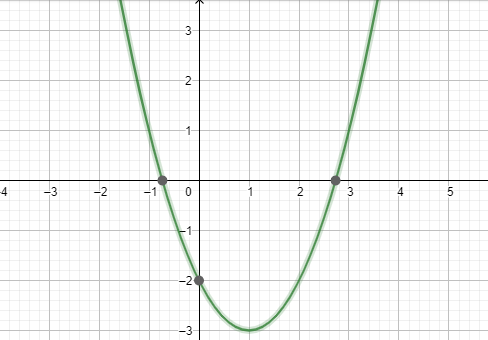## The Big Idea: What are Quadratic Equations?

Univariate means that the equations contain only 1 variable, the variable can be denoted by $${x, y}$$ or any other symbol of your choice. The highest degree of the variable in a quadratic equation is 2, which basically means that the variable is squared or raised to the power of 2. This distinguishes it from a linear equation which only has a degree of one.

So, quadratic equations are univariate algebraic expressions of degree two.

The standard form of a quadratic equation is:

$$ax^2+ bx + c = 0;$$

where a, b and c are nothing but numerical coefficients, you can replace them with any number and $${x}$$ is the variable.

You can clearly see here that the highest power of $${x}$$ is 2, which makes the equation a quadratic one (the word quadratic is derived from quad, meaning square). Another point to note over here is that if you keep a as 0, then the equation becomes a linear one and no longer remains a quadratic equation.

Have a look at how linear and quadratic equations differ graphically. This will allow you to get a better picture of the nature of these equations.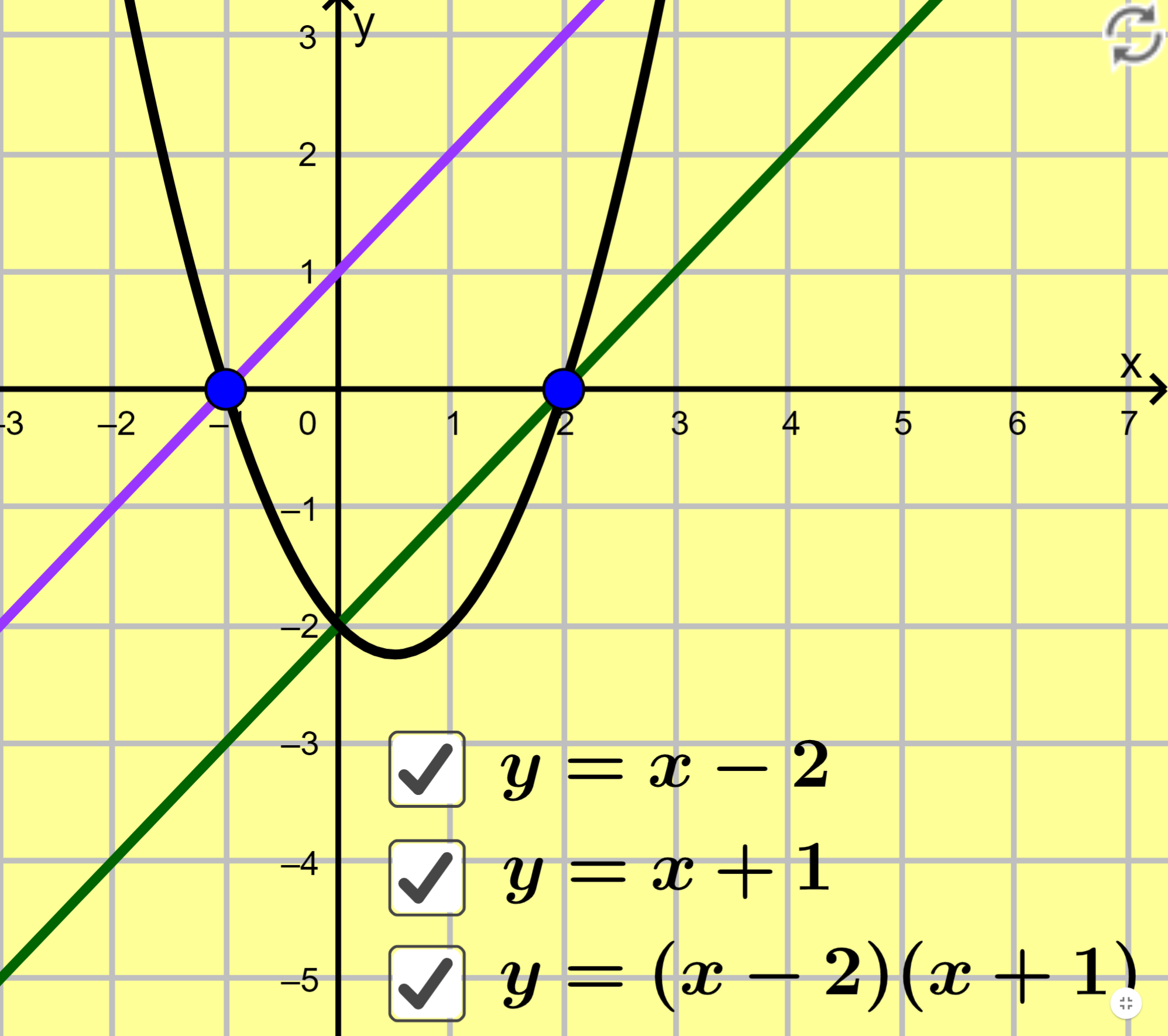### What is the Quadratic Formula and What does it mean?

When you see a quadratic equation, you can see that it cuts the $${x}$$-axis at 2 points at the most. These represent the Roots or Solutions to the equation. Let us take a simple quadratic equation to see its solutions or roots: $${x^2 – x – 2 = 0;}$$ now if we are to compare this equation with the standard equation, we can see that $${a = 1, \;b = - 1}$$ and $${c = - 2}$$.

Now, if we solve this equation for $${x}$$, we will see that there are 2 values of $${x}$$ which satisfy the equation: the 2 values are –1 and 2.

Let us represent this problem graphically to clearly see the curve and the solutions of the equation: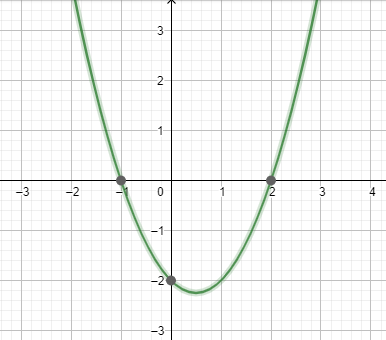If we were to solve the standard equation for $${x}$$, we get the quadratic formula given below: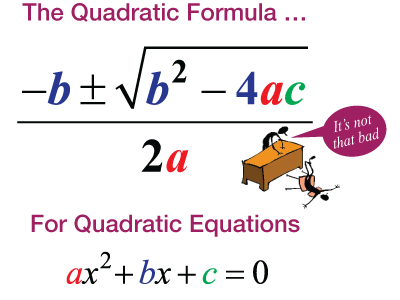The reason for the “plus/minus” sign is that a quadratic equation can have at the most 2 solutions. The 2 solutions are found out by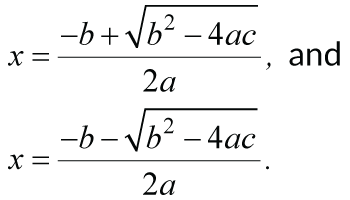For example, we solve the above equation $${x^2 – x – 2 = 0}$$ simply by replacing the values of $${a, \;b\; \text{and}\; c}$$ in the quadratic formula and get our solutions as –1 and 2. However, there are certain cases, where there may be less than 2 solutions to a particular quadratic equation. For example, there may only be 1 solution or there may be no solution at all. To understand it graphically, sometimes, the curve may touch the $${x}$$-axis only at one point or at times, it may not touch the $${x}$$-axis at all!

Thankfully though, we do not need to go about solving every equation to find out if it has 2, 1 or 0 solutions. We simply use, what is called, the Discriminant of the quadratic equation to find out the number of roots it has. The Discriminant (D) of a standard quadratic equation is nothing but the term under the square root of the quadratic formula.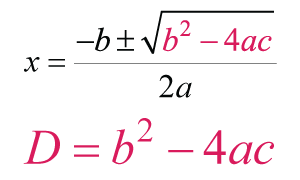The value of D determines the number of roots of the quadratic equation:

If
D > 0; the equation has 2 roots,
D = 0; the equation has 1 root and
D < 0; the equation has no roots

In higher grades, the concept of complex roots will be taught in case of D < 0, which involves an understanding of imaginary numbers.

Let us summarize the formulae at one place for a quick reference and recap:

Standard form of quadratic equation: $${ax2 + bx + c = 0;}$$
Solutions of a standard quadratic equation: $$x = {-b \pm \sqrt{b^2-4ac} \over 2a}$$

Discriminant (D) of a quadratic equation: $${b^2 – 4ac}$$

Conditions for the number of solutions of a quadratic equation:

D > 0; 2 Real roots,
D = 0; 1 Real root,
D < 0; 0 Real roots

## Tip and Trick:

A useful trick, which may come in handy while attempting questions related to quadratic equations is to first calculate the value of the discriminant, this eliminates the time taken in case the equation has no roots.